# Precalculus : Find the Area of a Triangle When Given One Side and Two Angles, Or When Given Two Sides and An Included Angle

## Example Questions

### Example Question #1 : Area Of A Triangle

In triangle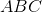,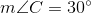,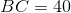, and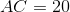.  Find the area of the triangle.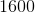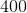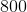Explanation:

When given the lengths of two sides and the measure of the angle included by the two sides, the area formula is: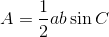Plugging in the given values we are able to calculate the area.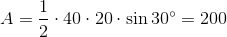### Example Question #46 : Trigonometric Applications

Find the area of this triangle: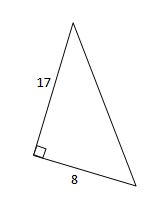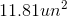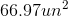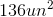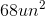Explanation:

To find the area, use the formula associated with side, angle, side triangles which states,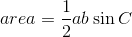whereandare side lengths andis the included angle.

In our case,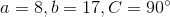.

Plug the values into the area formula and solve.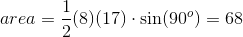### Example Question #47 : Trigonometric Applications

Find the area of this triangle: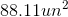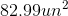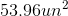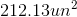Explanation:

Use the area formula to find area that is associated with the side angle side theorem for triangles.whereandare side lengths andis the included angle.

Plugging these values into the formula above, we arrive at our final answer.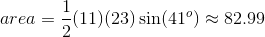### Example Question #48 : Trigonometric Applications

Find the area of this triangle: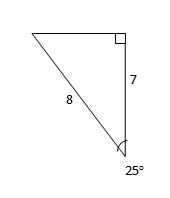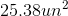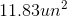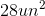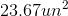Explanation:

To solve, use the formula for area that is associated with the side angle side theorem for triangles,whereandare side lengths andis the included angle.

Here we are using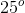and not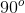since that is the angle betweenand.

Therefore,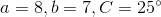.

Plugging the above values into the area formula we arrive at our final answer.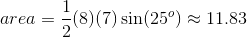### Example Question #1 : Find The Area Of A Triangle When Given One Side And Two Angles, Or When Given Two Sides And An Included Angle

Find the area of this triangle: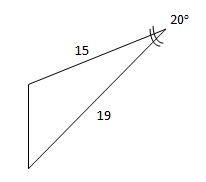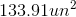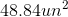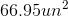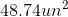Explanation:

Find the area using the formula associated the side angle side theorem of a triangle,whereandare side lengths andis the included angle.

In this particular case,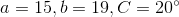therefore the area is found to be,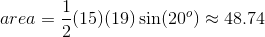.

### All Precalculus Resources